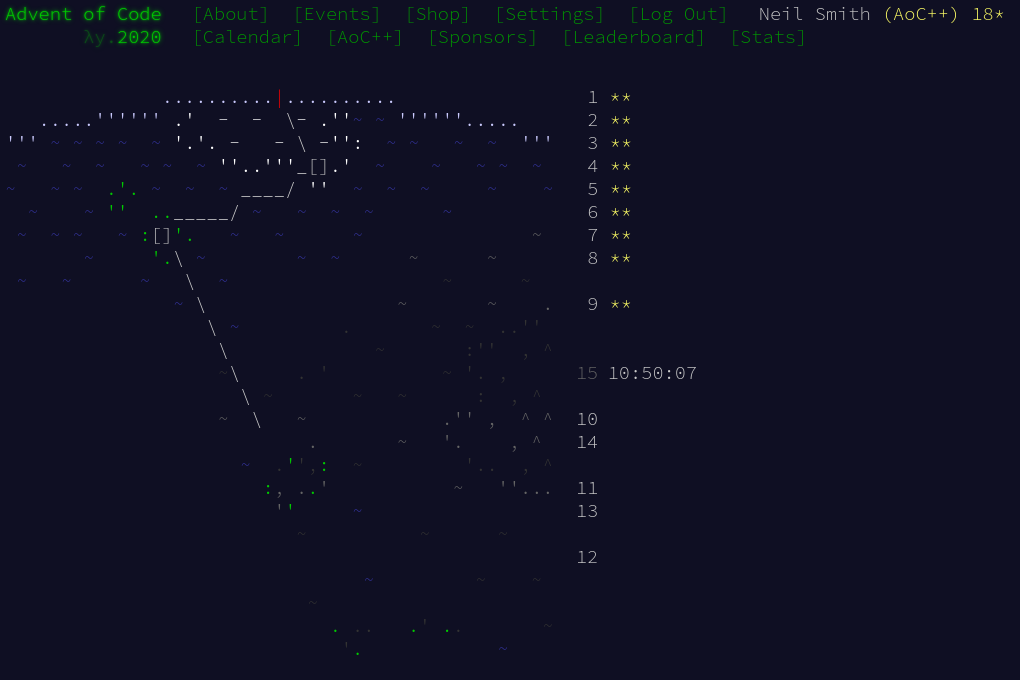December 15, 2020

# Advent of Code 2020 day 9Day 9 was a task that played very well to Haskell's strengths, using laziness for optimisation and a rich library of list-processing functions.

Workhorse functions are inits, tails, zip, and filter, all defined in Data.List.

## Part 1

I can create sliding window along a list by using map (take windowSize) $tails nums: tails creates all the starts of the sliding window, and the map (take windowSize) takes just the right number of elements to form the window. The result is a list of all the windows in the original list of nums. I then zip that with the list of numbers to be tested: zip (drop winSize nums) windows. That returns a list of (target, window) pairs. Given a window as a target, the test for validity follows the day 1 model of a list comprehension. part1 nums = fst$ head $filter (not . valid)$ slidingWindow 25 nums

slidingWindow winSize nums =
zip (drop winSize nums) $map (take winSize)$ tails nums

valid (target, window) = not $null [(x, y) | x <- window, y <- window, x + y == target]  ## Part 2 This revolves around finding all the substrings of the given list. Again, tails removes all the front parts of the list. inits removes all the back parts of the list. If I compose inits and tails, I get all the lists with some beginning and end removed. And given a list of numbers and a target, it's easy to tell if the list sums to the target. I use that predicate to filter all the subStrings. part2 target nums = (maximum section) + (minimum section) where section = head$ filter (sumsToTarget target) \$ subStrings smallNums
smallNums = dropWhileEnd (>= target) nums

subStrings = (concatMap inits) . tails

sumsToTarget target ns = sum ns == target


As a small efficiency gain, I noticed that the source list is generally (but not monotonically) increasing. There's no point examining the end of the source list if all the numbers there are larger than the target. dropWhileEnd handles that for me.

## Code

You can find the code here or on Gitlab.